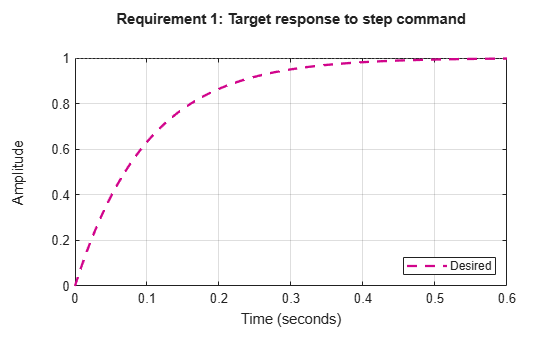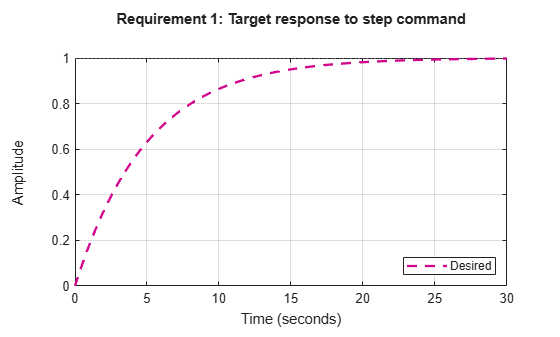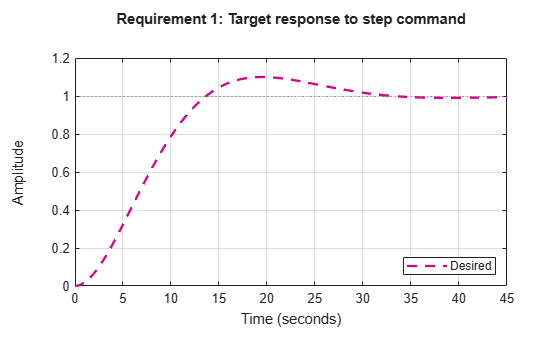# TuningGoal.StepTracking class

Package: TuningGoal

Step response requirement for control system tuning

## Description

Use `TuningGoal.StepTracking` to specify a target step response from specified inputs to specified outputs of a control system. Use this tuning goal with control system tuning commands such as `systune` or `looptune`.

## Construction

`Req = TuningGoal.StepTracking(inputname,outputname,refsys)` creates a tuning goal that constrains the step response between the specified signal locations to match the step response of a stable reference system, `refsys`. The constraint is satisfied when the relative difference between the tuned and target responses falls within a tolerance specified by the `RelGap` property of the tuning goal (see Properties). `inputname` and `outputname` can describe a SISO or MIMO response of your control system. For MIMO responses, the number of inputs must equal the number of outputs.

`Req = TuningGoal.StepTracking(inputname,outputname,tau)` specifies the desired step response as a first-order response with time constant `tau`:

`$\text{Req}\text{.ReferenceModel}=\frac{1/\text{tau}}{s+1/\text{tau}}.$`

`Req = TuningGoal.StepTracking(inputname,outputname,tau,overshoot)` specifies the desired step response as a second-order response with natural period `tau`, natural frequency 1/`tau`, and percent overshoot `overshoot`:

`$\text{Req}\text{.ReferenceModel}=\frac{{\left(1/\text{tau}\right)}^{2}}{{s}^{2}+2\left(\text{zeta}/\text{tau}\right)s+{\left(1/\text{tau}\right)}^{2}}.$`

The damping is given by ```zeta = cos(atan2(pi,-log(overshoot/100)))```.

### Input Arguments

 `inputname` Input signals for the tuning goal, specified as a character vector or, for multiple-input tuning goals, a cell array of character vectors. If you are using the tuning goal to tune a Simulink® model of a control system, then `inputname` can include:Any model input.Any linear analysis point marked in the model.Any linear analysis point in an `slTuner` (Simulink Control Design) interface associated with the Simulink model. Use `addPoint` (Simulink Control Design) to add analysis points to the `slTuner` interface. Use `getPoints` (Simulink Control Design) to get the list of analysis points available in an `slTuner` interface to your model. For example, suppose that the `slTuner` interface contains analysis points `u1` and `u2`. Use `'u1'` to designate that point as an input signal when creating tuning goals. Use `{'u1','u2'}` to designate a two-channel input. If you are using the tuning goal to tune a generalized state-space (`genss`) model of a control system, then `inputname` can include: Any input of the `genss` model Any `AnalysisPoint` location in the control system modelFor example, if you are tuning a control system model, `T`, then `inputname` can be any input name in `T.InputName`. Also, if `T` contains an `AnalysisPoint` block with a location named `AP_u`, then `inputname` can include `'AP_u'`. Use `getPoints` to get a list of analysis points available in a `genss` model.If `inputname` is an `AnalysisPoint` location of a generalized model, the input signal for the tuning goal is the implied input associated with the `AnalysisPoint` block:For more information about analysis points in control system models, see Mark Signals of Interest for Control System Analysis and Design. `outputname` Output signals for the tuning goal, specified as a character vector or, for multiple-output tuning goals, a cell array of character vectors. If you are using the tuning goal to tune a Simulink model of a control system, then `outputname` can include:Any model output.Any linear analysis point marked in the model.Any linear analysis point in an `slTuner` (Simulink Control Design) interface associated with the Simulink model. Use `addPoint` (Simulink Control Design) to add analysis points to the `slTuner` interface. Use `getPoints` (Simulink Control Design) to get the list of analysis points available in an `slTuner` interface to your model. For example, suppose that the `slTuner` interface contains analysis points `y1` and `y2`. Use `'y1'` to designate that point as an output signal when creating tuning goals. Use `{'y1','y2'}` to designate a two-channel output. If you are using the tuning goal to tune a generalized state-space (`genss`) model of a control system, then `outputname` can include: Any output of the `genss` model Any `AnalysisPoint` location in the control system modelFor example, if you are tuning a control system model, `T`, then `outputname` can be any output name in `T.OutputName`. Also, if `T` contains an `AnalysisPoint` block with a location named `AP_u`, then `outputname` can include `'AP_u'`. Use `getPoints` to get a list of analysis points available in a `genss` model.If `outputname` is an `AnalysisPoint` location of a generalized model, the output signal for the tuning goal is the implied output associated with the `AnalysisPoint` block:For more information about analysis points in control system models, see Mark Signals of Interest for Control System Analysis and Design. `refsys` Reference system for target step response, specified as a dynamic system model, such as a `tf`, `zpk`, or `ss` model. `refsys` must be stable and must have DC gain of 1 (zero steady-state error). `refsys` can be continuous or discrete. If `refsys` is discrete, it can include time delays which are treated as poles at `z` = 0. `refsys` can be MIMO, provided that it is square and that its DC singular value (`sigma`) is 1. If `refsys` is a MIMO model, then its number of inputs and outputs must match the dimensions of `inputname` and `outputname`. For best results, `refsys` should also include intrinsic system characteristics such as non-minimum-phase zeros (undershoot). `tau` Time constant or natural period of target step response, specified as a positive scalar. If you use the syntax ```Req = TuningGoal.StepTracking(inputname,outputname,tau)``` to specify a first-order target response, then `tau` is the time constant of the response decay. In that case, the target is the step response of the system given by: `$\text{Req}\text{.ReferenceModel}=\frac{1/\text{tau}}{s+1/\text{tau}}.$` If you use the syntax ```Req = TuningGoal.StepTracking(inputname,outputname,tau,overshoot)``` to specify a second-order target response, then `tau` is the inverse of the natural frequency of the response. In that case, the target is the step response of the system given by: `$\text{Req}\text{.ReferenceModel}=\frac{{\left(1/\text{tau}\right)}^{2}}{{s}^{2}+2\left(\text{zeta}/\text{tau}\right)s+{\left(1/\text{tau}\right)}^{2}}.$`The damping of the system is given by ```zeta = cos(atan2(pi,-log(overshoot/100)))```. `overshoot` Percent overshoot of target step response, specified as a scalar value in the range (0,100).

## Properties

 `ReferenceModel` Reference system for target step response, specified as a SISO or MIMO state-space (`ss`) model. When you use the tuning goal to tune a control system, the step response from `inputname` to `outputname` is tuned to match this target response to within the tolerance specified by the `RelGap` property. If you use the `refsys` input argument to create the tuning goal, then the value of `ReferenceModel` is `ss(refsys)`. If you use the `tau` or `tau` and `overshoot` input arguments, then`ReferenceModel` is a state-space representation of the corresponding first-order or second-order transfer function. `ReferenceModel` must be stable and have unit DC gain (zero steady-state error). For best results, `ReferenceModel` should also include intrinsic system characteristics such as non-minimum-phase zeros (undershoot). `RelGap` Maximum relative matching error, specified as a positive scalar value. This property specifies the matching tolerance as the maximum relative gap between the target and actual step responses. The relative gap is defined as: `$\text{gap}=\frac{{‖y\left(t\right)-{y}_{ref}\left(t\right)‖}_{2}}{{‖1-{y}_{ref}\left(t\right)‖}_{2}}.$` y(t) – yref(t) is the response mismatch, and 1 – yref(t) is the step-tracking error of the target model. ${‖\text{\hspace{0.17em}}\cdot \text{\hspace{0.17em}}‖}_{2}$ denotes the signal energy (2-norm). Increase the value of `RelGap` to loosen the matching tolerance. Default: 0.1 `InputScaling` Reference signal scaling, specified as a vector of positive real values. For a MIMO tracking requirement, when the choice of units results in a mix of small and large signals in different channels of the response, use this property to specify the relative amplitude of each entry in the vector-valued step input. This information is used to scale the off-diagonal terms in the transfer function from reference to tracking error. This scaling ensures that cross-couplings are measured relative to the amplitude of each reference signal. For example, suppose that `Req` is a tuning goal that signals `{'y1','y2'}` track reference signals `{'r1','r2'}`. Suppose further that you require the outputs to track the references with less than 10% cross-coupling. If `r1` and `r2` have comparable amplitudes, then it is sufficient to keep the gains from `r1` to `y2` and `r2` and `y1` below 0.1. However, if `r1` is 100 times larger than `r2`, the gain from `r1` to `y2` must be less than 0.001 to ensure that `r1` changes `y2` by less than 10% of the `r2` target. To ensure this result, set the `InputScaling` property as follows. `Req.InputScaling = [100,1];` This tells the software to take into account that the first reference signal is 100 times greater than the second reference signal. The default value, `[]` , means no scaling. Default: `[]` `Input` Input signal names, specified as a cell array of character vectors that identify the inputs of the transfer function that the tuning goal constrains. The initial value of the `Input` property is set by the `inputname` input argument when you construct the tuning goal. `Output` Output signal names, specified as a cell array of character vectors that identify the outputs of the transfer function that the tuning goal constrains. The initial value of the `Output` property is set by the `outputname` input argument when you construct the tuning goal. `Models` Models to which the tuning goal applies, specified as a vector of indices. Use the `Models` property when tuning an array of control system models with `systune`, to enforce a tuning goal for a subset of models in the array. For example, suppose you want to apply the tuning goal, `Req`, to the second, third, and fourth models in a model array passed to `systune`. To restrict enforcement of the tuning goal, use the following command: `Req.Models = 2:4;` When `Models = NaN`, the tuning goal applies to all models. Default: `NaN` `Openings` Feedback loops to open when evaluating the tuning goal, specified as a cell array of character vectors that identify loop-opening locations. The tuning goal is evaluated against the open-loop configuration created by opening feedback loops at the locations you identify. If you are using the tuning goal to tune a Simulink model of a control system, then `Openings` can include any linear analysis point marked in the model, or any linear analysis point in an `slTuner` (Simulink Control Design) interface associated with the Simulink model. Use `addPoint` (Simulink Control Design) to add analysis points and loop openings to the `slTuner` interface. Use `getPoints` (Simulink Control Design) to get the list of analysis points available in an `slTuner` interface to your model. If you are using the tuning goal to tune a generalized state-space (`genss`) model of a control system, then `Openings` can include any `AnalysisPoint` location in the control system model. Use `getPoints` to get the list of analysis points available in the `genss` model. For example, if `Openings = {'u1','u2'}`, then the tuning goal is evaluated with loops open at analysis points `u1` and `u2`. Default: `{}` `Name` Name of the tuning goal, specified as a character vector. For example, if `Req` is a tuning goal: `Req.Name = 'LoopReq';` Default: `[]`

## Examples

### Step Response Requirement with Specified Tolerance

Create a requirement for the step response from a signal named `'r'` to a signal named `'y'`. Constrain the step response to match the transfer function H = 10/(s+10), but allow 20% relative variation between the target the tuned responses.

```H = tf(10,[1 10]); Req = TuningGoal.StepResp('r','y',H);```

By default, this requirement allows a relative gap of 0.1 between the target and tuned responses. To change the relative gap to 20%, set the `RelGap` property of the requirement.

`Req.RelGap = 0.2;`

Examine the requirement.

`viewGoal(Req);`The dashed line shows the target step response specified by this requirement. You can use this requirement to tune a control system model, `T`, that contains valid input and output locations named `'r'` and `'y'`. If you do so, the command `viewGoal(Req,T)` plots the achieved step response from `'r'` to `'y'` for comparison to the target response.

### First-Order Step Response With Known Time Constant

Create a requirement that specifies a first-order step response with time constant of 5 seconds. Create the requirement for the step response from a signal named `'r'` to a signal named `'y'`.

`Req = TuningGoal.StepResp('r','y',5);`

When you use this requirement to tune a control system model, `T`, the time constant 5 is taken to be expressed in the prevailing units of the control system. For example, if `T` is a `genss` model and the property `T.TimeUnit` is `'seconds'`, then this requirement specifies a target time constant of 5 seconds for the response from the input `'r'` to the output `'y'` of `'T'`.

The specified time constant is converted into a reference state-space model stored in the `ReferenceModel` property of the requirement.

`refsys = tf(Req.ReferenceModel)`
```refsys = 0.2 ------- s + 0.2 Continuous-time transfer function. ```

As expected, `refsys` is a first-order model.

Examine the requirement. The `viewGoal` command displays the target response, which is the step response of the reference model.

`viewGoal(Req);`The dashed line shows the target step response specified by this requirement, a first-order response with a time constant of five seconds.

### Second-Order Step Response With Known Natural Period and Overshoot

Create a requirement that specifies a second-order step response with a natural period of 5 seconds, and a 10% overshoot. Create the requirement for the step response from a signal named `'r'` to a signal named `'y'`.

`Req = TuningGoal.StepResp('r','y',5,10);`

When you use this requirement to tune a control system model, `T`, the natural period 5 is taken to be expressed in the prevailing units of the control system. For example, if `T` is a `genss` model and the property `T.TimeUnit` is `'seconds'`, then this requirement specifies a target natural period of 5 seconds for the response from the input `'r'` to the output `'y'` of `'T'`.

The specified parameters of the response is converted into a reference state-space model stored in the `ReferenceModel` property of the requirement.

`refsys = tf(Req.ReferenceModel)`
```refsys = 0.04 --------------------- s^2 + 0.2365 s + 0.04 Continuous-time transfer function. ```

As expected, `refsys` is a second-order model.

Examine the requirement. The `viewGoal` command displays the target response, which is the step response of the reference model.

`viewGoal(Req);`The dashed line shows the target step response specified by this requirement, a second-order response with 10% overshoot and a natural period of five seconds.

### Tracking Goal with Limited Model Application and Additional Loop Openings

Create a tuning goal that specifies a first-order step response with time constant of 5 seconds. Set the `Models` and `Openings` properties to further configure the tuning goal’s applicability.

```Req = TuningGoal.StepTracking('r','y',5); Req.Models = [2 3]; Req.Openings = 'OuterLoop'```

When tuning a control system that has an input `'r'`, an output `'y'`, and an analysis-point location `'OuterLoop'`, you can use `Req` as an input to `looptune` or `systune`. Setting the `Openings` property specifies that the step response from `'r'` to `'y'` is measured with the loop opened at `'OuterLoop'`. When tuning an array of control system models, setting the `Models` property restricts how the tuning goal is applied. In this example, the tuning goal applies only to the second and third models in an array.

## Tips

• This tuning goal imposes an implicit stability constraint on the closed-loop transfer function from `Input` to `Output`, evaluated with loops opened at the points identified in `Openings`. The dynamics affected by this implicit constraint are the stabilized dynamics for this tuning goal. The `MinDecay` and `MaxRadius` options of `systuneOptions` control the bounds on these implicitly constrained dynamics. If the optimization fails to meet the default bounds, or if the default bounds conflict with other requirements, use `systuneOptions` to change these defaults.

## Algorithms

When you tune a control system using a `TuningGoal`, the software converts the tuning goal into a normalized scalar value f(x). Here, x is the vector of free (tunable) parameters in the control system. The software then adjusts the parameter values to minimize f(x) or to drive f(x) below 1 if the tuning goal is a hard constraint.

For `TuningGoal.StepTracking`, f(x) is given by:

`$f\left(x\right)=\frac{{‖\frac{1}{s}\left(T\left(s,x\right)-{T}_{ref}\left(s\right)\right)‖}_{2}}{\text{RelGap}{‖\frac{1}{s}\left({T}_{ref}\left(s\right)-I\right)‖}_{2}}.$`

T(s,x) is the closed-loop transfer function from `Input` to `Output` with parameter values x, and Tref(s) is the reference model specified in the `ReferenceModel` property. ${‖\text{\hspace{0.17em}}\cdot \text{\hspace{0.17em}}‖}_{2}$ denotes the H2 norm (see `norm`).

## Version History

Introduced in R2016a

expand all